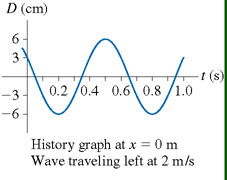# Problem: Part AWhat is the amplitude of this wave?Part BWhat is the frequency of this wave?Part CWhat is the wavelength of this wave?

###### FREE Expert Solution

Amplitude is the maximum displacement from the equilibrium position.

Frequency:

$\overline{){\mathbf{f}}{\mathbf{=}}\frac{\mathbf{1}}{\mathbf{T}}}$, where T is period.

The velocity of wave propagation:

$\overline{){\mathbf{v}}{\mathbf{=}}{\mathbf{f}}{\mathbf{\lambda }}}$, where f is frequency and λ is the wavelength.

93% (302 ratings)###### Problem Details

Part A

What is the amplitude of this wave?

Part B

What is the frequency of this wave?

Part C

What is the wavelength of this wave?Frequently Asked Questions

What scientific concept do you need to know in order to solve this problem?

Our tutors have indicated that to solve this problem you will need to apply the What is a Wave? concept. You can view video lessons to learn What is a Wave?. Or if you need more What is a Wave? practice, you can also practice What is a Wave? practice problems.